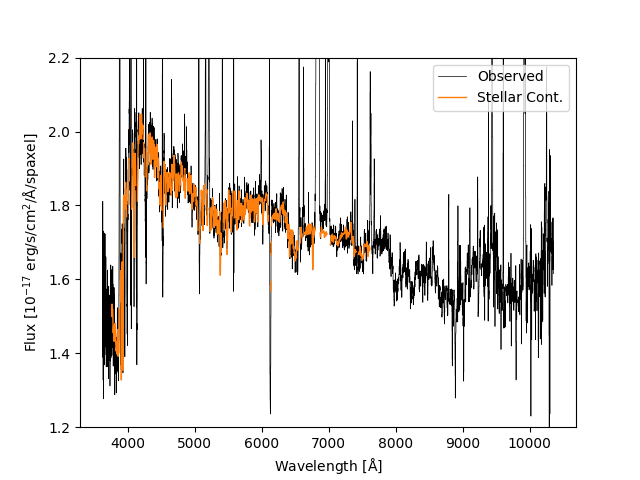# DAP Python Tutorial

Back to MaNGA tutorials

Disclaimer: This tutorial teaches you how to look at the DAP output files using standard python packages. However, we highly recommend you consider using Marvin, a python package designed specifically for downloading, visualizing, and analyzing MaNGA data.

The goal of this introductory tutorial will be to show you the basics of loading and visualizing MaNGA DAP products using python. Start by importing the basic packages we'll need:

import numpy as np
from astropy.io import fits
from matplotlib import pyplot as plt


Now, read in a maps file. For this tutorial, we'll use the maps file for MaNGA object (plate-ifu) 7443-12703, which you can download here.

# This assumes the MAPS file is in the current directory.  If that's not
# true, replace '' below with the directory containing the data file.
dir = ''


The MAPS file contains several extensions, which you can list using:

hdu.info()


For a full description of these extensions, see the MaNGA Data Model.

## Making an emission-line map and applying masks

Let's make a simple map of Hα flux. The Hα flux measurements are stored in the EMLINE_GFLUX extension. If we examine the size of the data in this extension:

hdu['EMLINE_GFLUX'].data.shape


we'll see that it is three dimensional; i.e., the shape is (35, 74, 74). The fits reader from Astropy reads the data ordered by "channel" number, y position, x position. Here, we refer to the first axis as the "channel" axis, where a "channel" provides the flux for a given emission line. We can see which channel corresponds to which emission line by looking at the header:

hdu['EMLINE_GFLUX'].header

This should show an entry that looks like C24 = 'Ha-6564 ' / Data in channel 24, indicating that the Hα flux is in channel 24. Channels are 1-indexed numbers, but python arrays are 0-indexed, meaning to get the Hα flux map we do the following:

flux_halpha = hdu['EMLINE_GFLUX'].data


Note that we've only provided the index of the first axis, which will correctly select the channel and return a 2D array. Particularly given the difference in the number of emission-lines measured in DR15/DR16 and DR17, it is better to select the channels based on the channel name. You can do this by making a dictionary that maps the different line names to their corresponding index as follows:

emline = {}
if k == 'C':
try:
i = int(k[1:])-1
except ValueError:
continue
emline[v] = i


So now we can select the Hα flux map using:

flux_halpha = hdu['EMLINE_GFLUX'].data[emline['Ha-6564']]


Now we can plot the map with a colorbar:

plt.clf()
plt.imshow(hdu['EMLINE_GFLUX'].data[emline['Ha-6564']], origin='lower', interpolation='nearest',
cmap='inferno')
plt.colorbar(label=r'H$\alpha$ Flux [$10^{-17}$ erg/s/cm$^2$/spaxel]')
plt.show()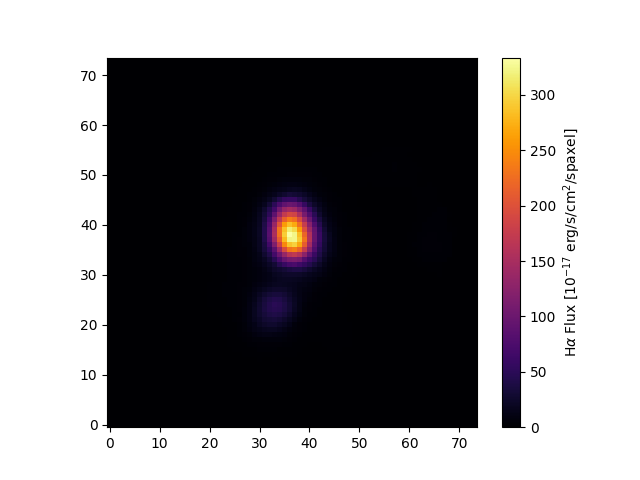Unmasked Hα flux map for 7443-12703.

Typically, the maps contain some spaxels with unreliable measurements. The MAPS files contains bitmask extensions that must be used to identify these spaxels. The header of the EMLINE_GFLUX extension tells you which extension holds its mask.

mask_extension = hdu['EMLINE_GFLUX'].header['QUALDATA']


We can use this extension to make a masked image

masked_image = np.ma.array(hdu['EMLINE_GFLUX'].data[emline['Ha-6564']],
plt.clf()
plt.colorbar(label=r'H$\alpha$ Flux [$10^{-17}$ erg/s/cm$^2$/spaxel]')
plt.show()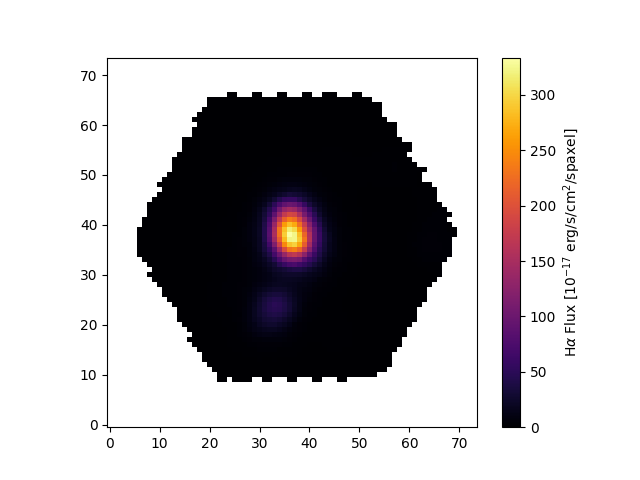Masked Hα flux map for 7443-12703.

Note that, compared to the previous figure, we can see the outline of the hexagonal pattern of the MaNGA IFU; i.e., the mask has selected only those spaxels with coverage by the MaNGA IFU.

Now, let's use the same basic procedure to plot the ionized-gas velocity field.

mask_ext = hdu['EMLINE_GVEL'].header['QUALDATA']
plt.clf()
plt.imshow(gas_vfield, origin='lower', interpolation='nearest', vmin=-125, vmax=125,
cmap='RdBu_r')
plt.colorbar(label=r'H$\alpha$ Velocity [km/s]')
plt.show()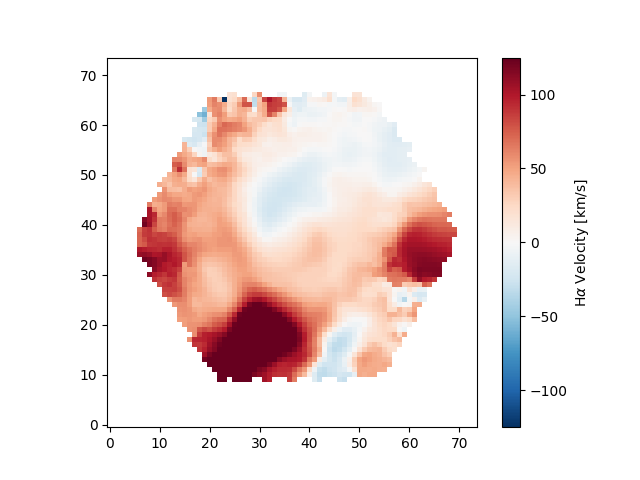Ionized-gas velocity field for 7443-12703.

Depending on your science, you may want to only examine regions with very well-detected emission lines. You can do this by masking data with low S/N using the provided flux uncertainties, which are given as inverse variances. We can find the proper extension with

ivar_extension = hdu['EMLINE_GFLUX'].header['ERRDATA']


Let's now calculate the S/N (here defined as flux over flux error) per spaxel and use it to reject spaxels where the line flux has S/N < 10

snr_map = hdu['EMLINE_GFLUX'].data[emline['Ha-6564']] \
* np.sqrt(hdu[ivar_extension].data[emline['Ha-6564']])
sncut = 10
| (snr_map[:,:] < sncut))
plt.clf()
plt.imshow(gas_vfield_alt, origin='lower', interpolation='nearest', vmin=-125, vmax=125,
cmap='RdBu_r')
plt.colorbar(label=r'H$\alpha$ Velocity [km/s]')
plt.show()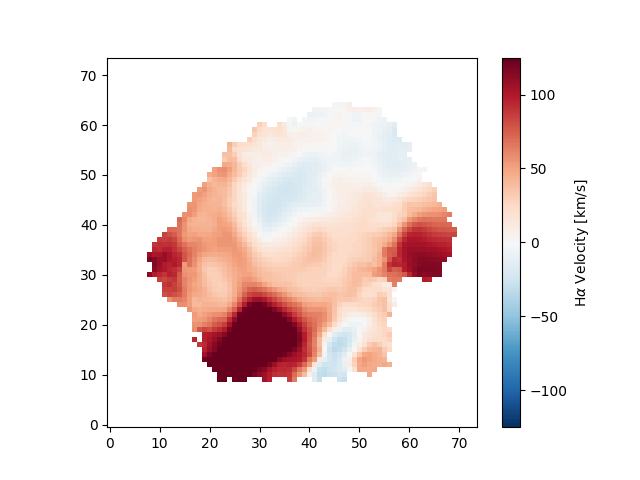Ionized-gas velocity field for 7443-12703, restricted to spaxels where the Hα flux has S/N>10.

An important note regarding gas velocity fields: The velocities of the emission lines are tied together, so for example, the velocity of the [OIII]-5007 line is the same as the Hα line, as are the uncertainties. You cannot reduce the uncertainty on the measured velocity by averaging the velocities of several lines together!

## Plotting stellar velocity dispersion

Next we'll make a map of the stellar velocity dispersion. First, we'll access the raw dispersion measurements:

disp_raw = hdu['STELLAR_SIGMA'].data


However, we need to correct the measured dispersion for the instrumental resolution, which is reported in a different extension":

disp_inst = hdu['STELLAR_SIGMACORR'].data


Note that, compared to DR15/DR16, we're required to select the first channel of the STELLAR_SIGMACORR extension in DR17. This extension now provides two extensions with different methods used to calculate the correction. You should always use the correction in the first channel.

Now, let's apply the correction and plot the results (also removing masked values). The calculation below will ignore any points where the correction is larger than the measured dispersion; see the discussion in Section 7.7 of Westfall et al. (2019, AJ, 158, 231) for specific recommendations for how to treat these data:

disp_stars_corr = np.ma.sqrt(disp_raw**2 - disp_inst**2)
plt.clf()
plt.imshow(disp_stars_corr, origin='lower', interpolation='nearest', cmap='viridis')
plt.colorbar(label=r'$\sigma_\ast$ [km/s]')
plt.show()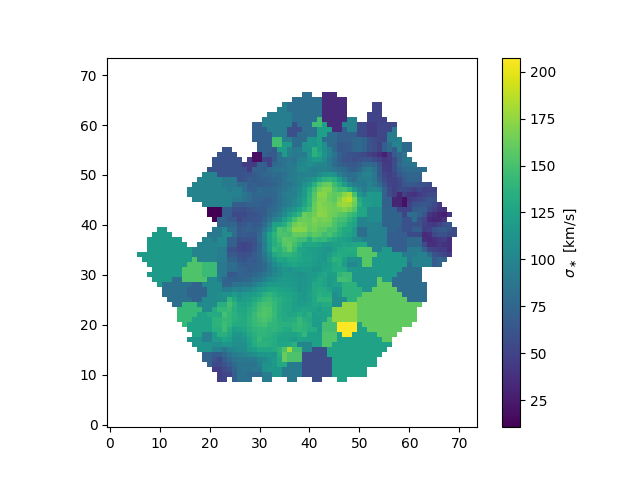Corrected stellar velocity dispersion map for 7443-12703.

## Plotting spectral-index measurements

Next we'll show how to plot spectral indices and correct them for velocity dispersion. In DR17, the DAP provides two versions of the spectral index measurements (found in extensions SPECINDEX and SPECINDEX_BF) that follow slightly different definitions; see more discussion here. Like the emission-line measurements, we can create a dictionary that makes accessing different spectral indices easier, as well as use the header information to determine the index units:

speci = {}
specu = np.empty(np.shape(hdu['SPECINDEX'].data),dtype=object)
if k == 'C':
try:
i = int(k[1:])-1
except ValueError:
continue
speci[v] = i
if k == 'U':
try:
i = int(k[1:])-1
except ValueError:
continue
specu[i] = v.strip()


We can now construct a masked array with the Hβ spectral index values:

mask_ext = hdu['SPECINDEX'].header['QUALDATA']


The spectral-index measurements should be corrected for velocity dispersion; i.e., to compare line strengths for galaxies with different stellar velocity dispersion, you must remove the effects of the velocity dispersion on the line strength. The correction method depends on the units; see discussion here. The Hβ index is measured in Angstroms, meaning the correction is multiplicative:

hb_corr = hb_raw*hdu['SPECINDEX_CORR'].data[speci['Hb']]
plt.clf()
plt.imshow(hb_corr,origin='lower', cmap='inferno', interpolation='nearest', vmin=-2, vmax=10)
plt.colorbar(label=specu[speci['Hb']])
plt.show()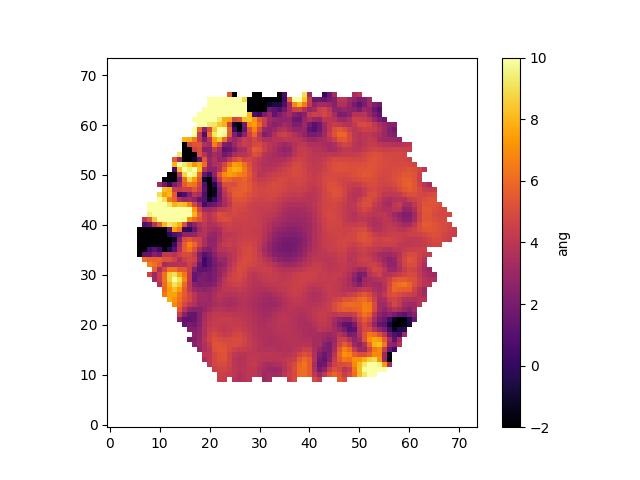Corrected Hβ spectral index map for 7443-12703.

## Identifying unique bins

The spaxels are binned in different ways depending on the measurement being made (the DAP documentation provides more information). This binning means that two spaxels can belong to the same bin, and therefore a derived quantity at those locations will be identical. The BINID extension provides information about which spaxels are in which bins; see more information here.

For any analysis, you'll want to extract the unique spectra and/or maps values. For example, to find the indices of the unique bins where stellar kinematics were fit:

bin_indx = hdu['BINID'].data
unique_bins, unique_indices = tuple(map(lambda x : x[1:],
np.unique(bin_indx.ravel(), return_index=True)))


The first line select the BINID values specifically for those used for the stellar kinematics, and the second line uses the numpy unique method to find the first occurrence of the unique bin ID numbers. The removal of the first unique value is just to remove the ever-present BINID=-1 values (i.e., all MaNGA MAPS files have spaxels with BINID=-1 given the hexagonal shape of the IFU and the square shape of the MAPS).
Let's now use this information to plot the position of each unique bin and color-code it by the measured stellar velocity:

# Get the luminosity-weighted x and y coordinates of the unique bins
x = hdu['BIN_LWSKYCOO'].data.ravel()[unique_indices]
y = hdu['BIN_LWSKYCOO'].data.ravel()[unique_indices]
#  ... and the measured stellar velocities
# Use a scatter plot to show the velocity field
plt.clf()
plt.scatter(x, y, c=v, vmin=-150, vmax=150, cmap='seismic', marker='.', s=30, lw=0, zorder=3)
plt.colorbar(label=r'$V_\ast$ [km/s]')
plt.show()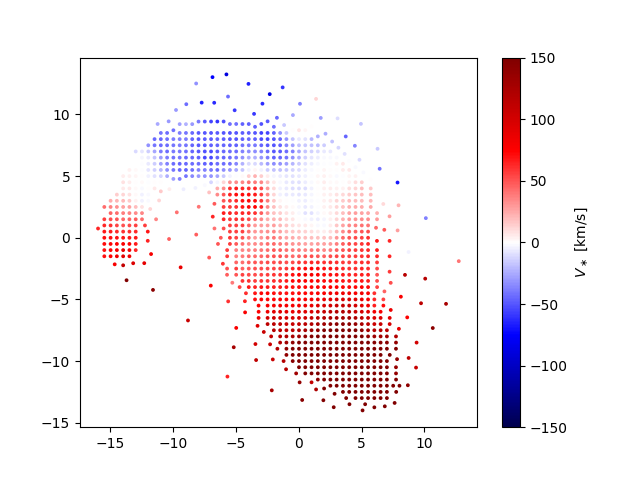Positions of each unique stellar velocity measurements for the binned spectra in 7443-12703, color-coded by value.

## Extract a binned spectrum and its model fit

The model cube files provide the binned spectra and the best-fit models. We can use these files to compare an individual bin's measured spectrum, model fit, model residuals, and so on. However, one needs to be careful when comparing binned spectra with the model fits. Specifically, there are three types of files with different binning schemes:

• SPX: All spaxels with S/N ≥ 1 are analyzed.
• VOR10: The spectra are Voronoi binned to S/N ˜ 10. Stellar and emission line parameters are estimated from those bins.
• HYB10: The spectra are again Voronoi binned to S/N ˜ 10, and the stellar parameters are calculated using these Voronoi binned spectra. However, emission-line parameters are measured using the individual 0.5"x0.5" spaxels.

If you are using the HYB10 files and want to compare the best-fitting model (including stellar continuum and emission lines) to the data, you need to compare the models to the individual spectra (measured in 0.5"x0.5" spaxels) from the DRP LOGCUBE files, not the binned spectra in the HYB10 files. If you are comparing the best-fitting stellar-continuum models to the data, you should use the binned spectra within the HYB10 files.

Below are a few examples using both VOR10 and HYB10 files to demonstrate the proper way to compare the models and data using these two files types.

### VOR10 Files

We'll start with the VOR10 files where comparing the models and data is simpler because all the spectra and models are for the same binned spectra. We'll first read in the BIN_SNR extension from the VOR10 maps file and find the bin with the highest S/N. The file for this example can be downloaded here.

hdu = fits.open(dir+'manga-7443-12703-MAPS-VOR10-MILESHC-MASTARSSP.fits.gz')
j,i = np.unravel_index(snr.argmax(), snr.shape)


Next we'll load the model cube file for this galaxy (can be downloaded here, pull out the relevant spectra for the highest S/N binned spectrum, and then plot the data, models, and residuals.

hdu_dapcube = fits.open(dir+'manga-7443-12703-LOGCUBE-VOR10-MILESHC-MASTARSSP.fits.gz')
wave = hdu_dapcube['WAVE'].data
resid = flux-model-0.5

plt.clf()
plt.step(wave, flux, where='mid', color='k', lw=0.5, label='Observed')
plt.plot(wave, model, color='C3', lw=1, label='Model')
plt.plot(wave, stellarcontinuum, color='C1', lw=1, label='Stellar Cont.')
plt.plot(wave, emlines, color='C0', lw=1, label='Emission Lines')
plt.step(wave, resid, where='mid', color='0.5', lw=0.5, label='Residual')
plt.xlabel(r'Wavelength [${\rm \AA}$]')
plt.ylabel(r'Flux [$10^{-17}$ erg/s/cm$^2$/${\rm \AA}$/spaxel]')
plt.legend()
plt.show()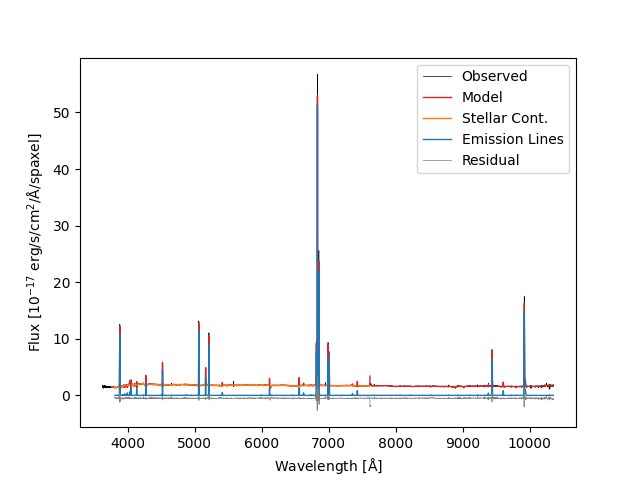Fit to the highest S/N bin in 7443-12703. Lines show the binned flux, full model fit, model stellar continuum, model emission lines, and model fit residuals.

### HYB10 Files

Identifying the bin with the highest S/N is done the same way as for the VOR10 files.

#DAP MAPS file
j,i = np.unravel_index(snr.argmax(), snr.shape)


Recall that although stellar parameters are measured using the Voronoi bins, the emission-line parameters are estimated using the individual spaxels. Therefore, if we want to compare the data to the full best-fitting model, which includes stellar continuum and emission lines, we need to use the spectra from the DRP LOGCUBE file, not the DAP HYB10 LOGCUBE file. Let's compare the best-fitting model to the data at the position (j,i) found above (DAP HYB10 LOGCUBE can be downloaded here):

#DAP MODELCUBE file
#DRP LOGCUBE file
hdu_drpcube = fits.open(dir+'manga-7443-12703-LOGCUBE.fits.gz')
# NOTE: the wavelength vectors for the DAP and DRP LOGCUBE files should
# be identical
wave = hdu_drpcube['WAVE'].data
plt.clf()
plt.step(wave, flux, where='mid', color='k', lw=0.5, label='Observed')
plt.plot(wave, model, color='C3', lw=1, label='Model')
plt.xlabel(r'Wavelength [${\rm \AA}$]')
plt.ylabel(r'Flux [$10^{-17}$ erg/s/cm$^2$/${\rm \AA}$/spaxel]')
plt.legend()
plt.show()Model fit from the HYB10 output for the highest S/N bin in 7443-12703.

If we just want to compare the best-fitting stellar continuum to the data, we should use the binned spectra within the DAP HYB10 LOGCUBE file:

binned_flux = np.ma.MaskedArray(hdu_dapcube['FLUX'].data[:,j,i],
plt.xlabel(r'Wavelength [${\rm \AA}$]')
plt.ylabel(r'Flux [$10^{-17}$ erg/s/cm$^2$/${\rm \AA}$/spaxel]')